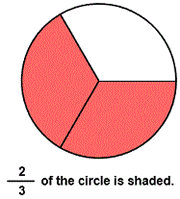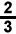# Identify fractions with circle models

## Circle models show the numerator and denominator to give instruction and practice in identifying fractions.

IDENTIFY FRACTIONS
CORRECT:
ATTEMPTS:
SCORE:
PERCENT
NUMERATOR
DENOMINATOR

# IDENTIFY FRACTIONS WITH CIRCLE MODELS INSTRUCTIONS

Follow the directions in the dialog box after pressing the <START> button. The <EXPLAIN> button may be pressed to see how to do the example.

Identify Fractions uses circles to demonstrate the meaning of numerator and denominator.

The following illustration was made by :The full circle a unit. This unit is divided into 3 equal parts. If we take the two colored parts, we have taken two of the three equal parts.

The top number 2 in the numeralis the numerator. The numerator tells us how many of the parts in the unit are to be taken.

The bottom number 3 in the numeralis the denominator. The denominator tells us the total number of equal parts into which the unit is divided. In this example there are 3 equal parts in the circle.

The line between the numerator and denominator is known as the fraction bar. It is also called the division bar.

You will enter the numeralfor te fraction because 2 of the 3 parts in the circle are colored.

For more instruction on identifying fractions go to How to Identify Fractions.

After you enter the fraction form answer you may press the <REPORT> button. The report will ask for your name but you may submit a code for your name. This report will give same results as on the dialog box. The report may be printed or e-mailed.